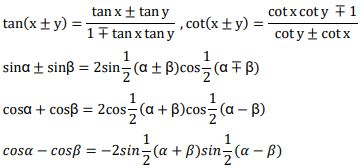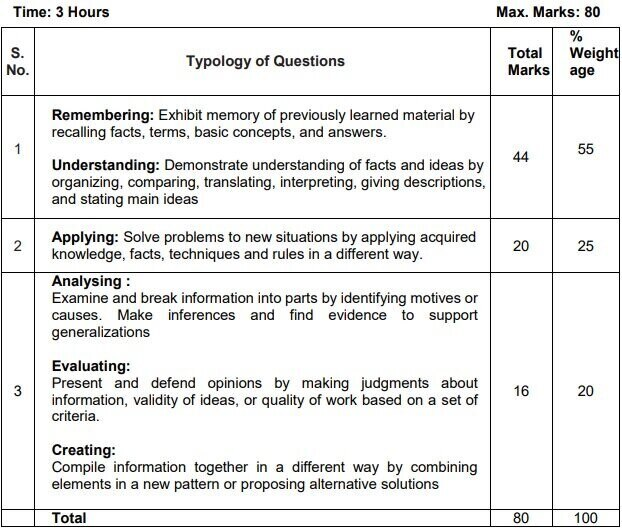# CBSE Class 11 Mathematics Syllabus 2022-2023 (New): Download Revised Curriculum in PDF

0
240CBSE Class 11 Maths Syllabus 2022-2023 is provided here for download in PDF. Students must check this revised and reduced syllabus to know the course content to be studied during the 2022-2023 session. This syllabus is set according to the annual scheme of assessment and the annual CBSE Class 11 Maths Exam will be based on the revised syllabus only. Therefore, students must go through the full syllabus and study Maths as per the content mentioned in it.

Check CBSE Class 11 Maths Course Structure 2022-23 below:

One Paper

Total Periods – 240 [35 Minutes each]

 Time: 3 Hours                                                                                Max Marks: 80 No. Units No. of Periods Marks I Sets and Functions 60 23 II Algebra 50 25 III Coordinate Geometry 50 12 IV Calculus 40 08 V Statistics and Probability 40 12 Total 240 80 Internal Assessment 20

*No chapter/unit-wise weightage. Care to be taken to cover all the chapters.

Unit-I: Sets and Functions

1.Sets (20 Periods)

Sets and their representations, Empty set, Finite and Infinite sets, Equal sets, Subsets, Subsets of a set of real numbers especially intervals (with notations). Universal set. Venn diagrams. Union and Intersection of sets. Difference of sets. Complement of a set. Properties of Complement.

2.Relations & Functions (20 Periods)

Ordered pairs. Cartesian product of sets. Number of elements in the Cartesian product of two finite sets. Cartesian product of the set of reals with itself (upto R x R x R).Definition of relation, pictorial diagrams, domain, co-domain and range of a relation. Function as a special type of relation. Pictorial representation of a function, domain, co-domain and range of a function. Real valued functions, domain and range of these functions, constant, identity, polynomial, rational, modulus, signum, exponential, logarithmic and greatest integer functions, with their graphs. Sum, difference, product and quotients of functions.

3.Trigonometric Functions (20 Periods)

Positive and negative angles. Measuring angles in radians and in degrees and conversion from one measure to another. Definition of trigonometric functions with the help of unit circle. Truth of the identity sin2x + cos2x = 1, for all x. Signs of trigonometric functions. Domain and range of trigonometric functions and their graphs. Expressing sin (x±y) and cos (x±y) in terms of sinx, siny, cosx & cosy and their simple applications. Deducing identities like the following:Identities related to sin2x, cos2x, tan2 x, sin3x, cos3x and tan3x.

Unit-II: Algebra

1.Complex Numbers and Quadratic Equations (10 Periods)

Need for complex numbers, especially √−1, to be motivated by inability to solve some of the quadratic equations. Algebraic properties of complex numbers. Argand plane

2.Linear Inequalities (10 Periods)

Linear inequalities. Algebraic solutions of linear inequalities in one variable and their representation on the number line.

3.Permutations and Combinations (10 Periods)

Fundamental principle of counting. Factorial n. (n!) Permutations and combinations, derivation of Formulae for nPr and nCr and their connections, simple applications.

4.Binomial Theorem (10 Periods)

Historical perspective, statement and proof of the binomial theorem for positive integral indices. Pascal’s triangle, simple applications.

5.Sequence and Series (10 Periods)

Sequence and Series. Arithmetic Mean (A.M.) Geometric Progression (G.P.), general term of a G.P., sum of n terms of a G.P., infinite G.P. and its sum, geometric mean (G.M.), relation between A.M. and G.M.

Unit-III: Coordinate Geometry

1.Straight Lines (15 Periods)

Brief recall of two dimensional geometry from earlier classes. Slope of a line and angle between two lines. Various forms of equations of a line: parallel to axis, point -slope form, slope-intercept form, two-point form, intercept form, Distance of a point from a line.

2.Conic Sections (25 Periods)

Sections of a cone: circles, ellipse, parabola, hyperbola, a point, a straight line and a pair of intersecting lines as a degenerated case of a conic section. Standard equations and simple properties of parabola, ellipse and hyperbola. Standard equation of a circle.

3.Introduction to Three-dimensional Geometry (10 Periods)

Coordinate axes and coordinate planes in three dimensions. Coordinates of a point. Distance between two points.

Unit-IV: Calculus

1.Limits and Derivatives (40 Periods)

Derivative introduced as rate of change both as that of distance function and geometrically. Intuitive idea of limit. Limits of polynomials and rational functions trigonometric, exponential and logarithmic functions. Definition of derivative relate it to scope of tangent of the curve, derivative of sum, difference, product and quotient of functions. Derivatives of polynomial and trigonometric functions.

Unit-V Statistics and Probability

1.Statistics (20 Periods)

Measures of Dispersion: Range, Mean deviation, variance and standard deviation of ungrouped/grouped data.

2.Probability (20 Periods)

Events; occurrence of events, ‘not’, ‘and’ and ‘or’ events, exhaustive events, mutually exclusive events, Axiomatic (set theoretic) probability, connections with other theories of earlier classes. Probability of an event, probability of ‘not’, ‘and’ and ‘or’ events.

MATHEMATICS QUESTION PAPER DESIGN CLASS – XI (2022-23)1.No chapter wise weightage. Care to be taken to cover all the chapters

2.Suitable internal variations may be made for generating various templates keeping the overall weightage to different form of questions and typology of questions same.

Choice(s):

There will be no overall choice in the question paper.

However, 33% internal choices will be given in all the sections

 INTERNAL ASSESSMENT 20 Marks Periodic Tests ( Best 2 out of 3 tests conducted) 10 Marks Mathematics Activities 10 Marks

Prescribed Books:

Mathematics Textbook for Class XI, NCERT Publications